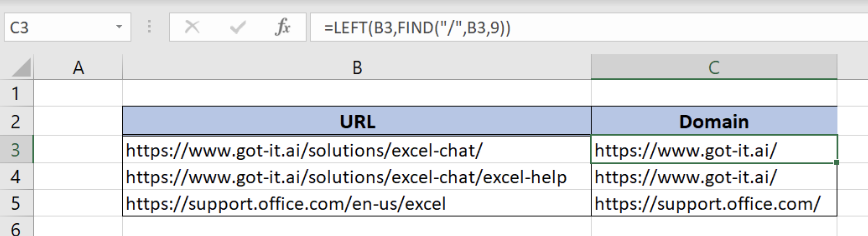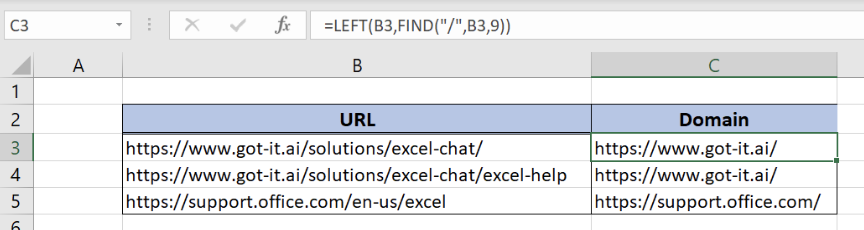Get instant live expert help with Excel or Google Sheets“My Excelchat expert helped me in less than 20 minutes, saving me what would have been 5 hours of work!”

#### Post your problem and you’ll get Expert help in seconds.

Your message must be at least 40 characters
Our professional Expert are available now. Your privacy is guaranteed.

# How to Get a Domain Name from a URL in Excel

In this tutorial, we will learn how to get domain name from URL in Excel. In order to do this, we will use LEFT and FIND functions. Combination of these two functions allows us to get a number of characters to the specific certain character.Figure 1. Final result

## Syntax of LEFT and FIND function

The generic formula to get domain name from URL is:

`=LEFT(text ,FIND(find_text,within_text,start_num))`

In order to get domain name from URL, we will have to use LEFT and FIND functions. Therefore, we will first explain both functions separately.

FIND function returns a position of a specific text in a cell.

The parameters of this function are:

• find_text – a text which we want to find in a cell
• within_text – a text cell in which we want to find a position of a character
• start_num – a position (character number) from which we want to look up for a text. This is a non-mandatory parameter.

LEFT function returns a certain number of characters from the left side in a cell.

The parameters of the function are:

• text – a cell from which we want to take substring
• num_chars – a number of characters which we want to take from the left side. This is a non-mandatory parameter.

Finally, The result of the FIND function will be a num_chars parameter for the LEFT function.Figure 2. The structure of the data

We will now explain the example. Let’s start with examining the structure of the data that we will use.

In column B (“URL”) we have URL, while in column C (“Domain”) we want to get domain from URL. A domain is a link from the beginning until the third “/”.Figure 3. Get domain name from URL

# Retrieve Domain Name from URL

The formula looks like:

`=LEFT(B3,FIND("/",B3,9))`

We will first evaluate the FIND part of the function, because we want to find the position of “/” in B3 cell beginning from the 9th character in B3. This is because the first two occurrences of “/” in B3 are included in the domain, while the third one is the end of the domain. Therefore, the result of `FIND("/",B3,9)` is 22.

This result will be the num_chars parameter of the LEFT function. So, we will have `=LEFT(B3,22)` function and take 22 characters from the left from B3, which represents the domain.

Above all, to get domain name from URL, we need to follow these steps:

• Select cell C3 and click on it
• Insert the formula `=LEFT(B3,FIND("/",B3,9))`
• Press enter
• Drag the formula down to the other cells in the column by clicking and dragging the little “+” icon at the bottom-right of the cell

As a result, we will get “https://www.got-it.ai/” in cell C3 for URL “https://www.got-it.ai/solutions/excel-chat/” from cell B3.

Most of the time, the problem you will need to solve will be more complex than a simple application of a formula or function. If you want to save hours of research and frustration, try our live Excelchat service! Our Excel Experts are available 24/7 to answer any Excel question you may have. We guarantee a connection within 30 seconds and a customized solution within 20 minutes.

### Did this post not answer your question? Get a solution from connecting with the expert.Another blog reader asked this question today on Excelchat:
Solution examplesI need to create a formula that extracts the prefix from column A
Solved by I. Q. in 42 minsi need a formula to combine column y with the number mentioned in column w counting in from 1 to 0 and posting the total count next to number 1 in column v.
Solved by E. J. in 60 minsI would like to insert a formula to add something (Maybe a 'Y') if a cell contains 14 days or less. Is this possible?
Solved by F. H. in 6 minsWhat is the formula for splitting up one column text into 2 columns?
Solved by B. H. in 20 minsI have text and number in multiple cells (Ex: Cell 1: 4S, Cell 2: 3.5A, Cell 3: 8O) and want to sum the number values only (Ex: 4 + 3.5 + 8). How can I do this?
Solved by O. A. in 60 mins## Subscribe to Excelchat.coAnother blog reader asked this question today on Excelchat: